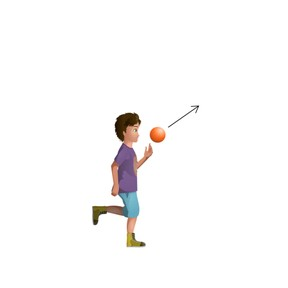Gravity and motion: Projectile motion

# Gravity and motion: Projectile motion

I can describe and predict the projectile motion of an object in two dimensions.8,000 schools use Gynzy92,000 teachers use Gynzy1,600,000 students use Gynzy

## General

In this lesson, students will build upon their knowledge of gravity and motion and look at how they interact in two dimensions.

NGSS:
MS-PS2-2
MS-PS2-4

## Learning objective

Students will be able to describe and predict the projectile motion of an object in two dimensions.

## Introduction

Students will be presented with a scenario that a boy throws a ball in an upward direction. Discuss with students the path the ball will follow. Review acceleration.

## Instruction

Students will imagine that they are out in space (without gravity) and push a ball from left to right. Go over horizontal motion, movement that runs parallel to the ground that is not influenced by gravity and has a constant velocity. Explain that when horizontal and vertical motion come together, the resulting motion is called projectile motion. Have students come up with a few examples of projectile motion. Show students this scenario: The tank on the cliff is trying to hit the target below. Its first shot came up a bit short. What can the tank do to hit the target next time?

Next, explain that orbit is when an object is moving in projectile motion along the curve of the earth. Review the concept of free fall.

## Quiz

Students will respond to ten multiple-choice and true/false questions.

## Closing

Close the lesson with a two-question exit slip.

### The online teaching platform for interactive whiteboards and displays in schools

• Save time building lessons

• Manage the classroom more efficiently

• Increase student engagement